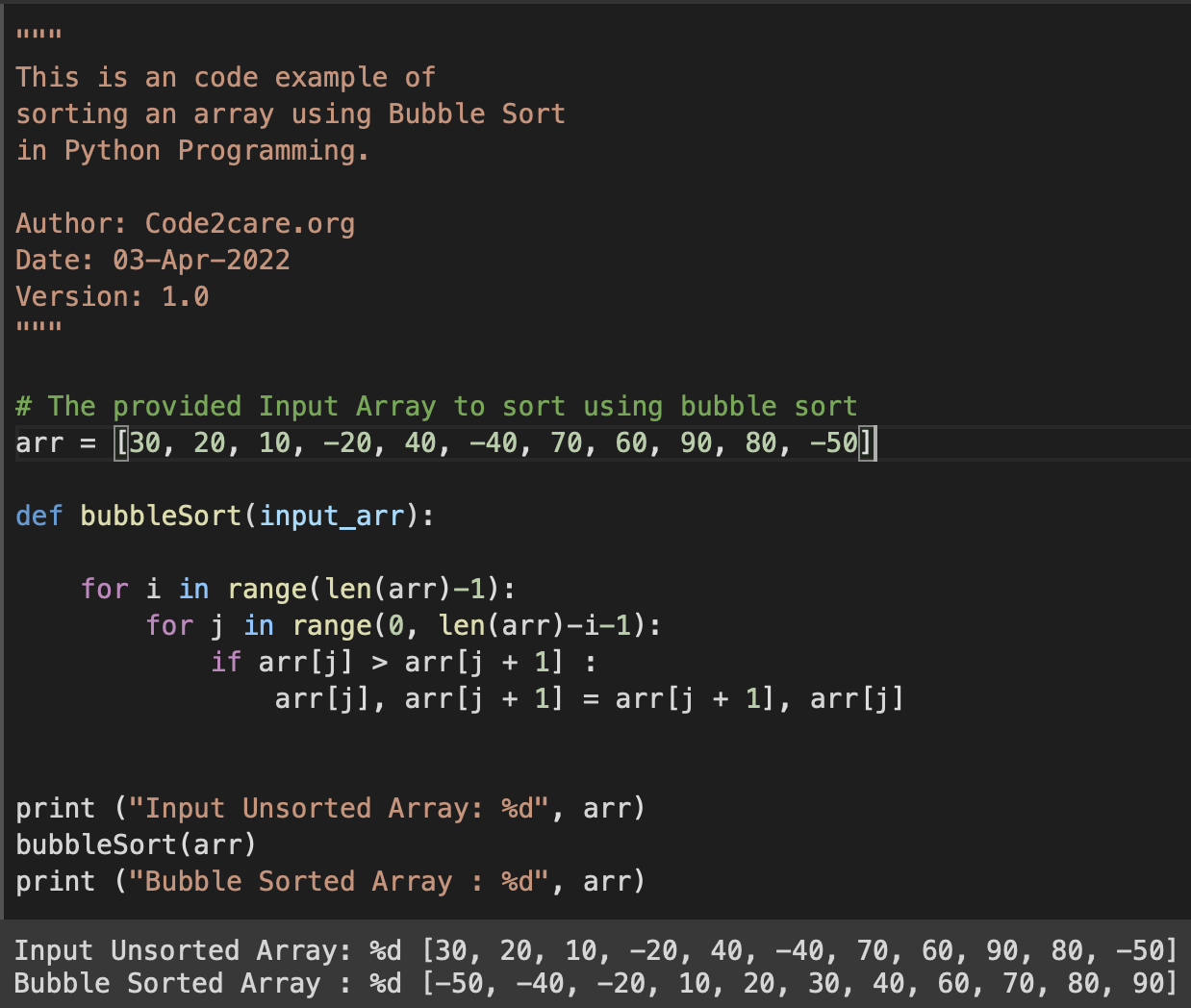# Sorting an array using Bubble Sort in Python Programming

Program for sorting an array in Python using Bubble Sort. Code:
``````"""
This is a code example of
sorting an array using Bubble Sort
in Python Programming.

Author: Code2care.org
Date: 03-Apr-2022
Version: 1.0
"""

# The provided Input Array to sort using bubble sort
arr = [30, 20, 10, -20, 40, -40, 70, 60, 90, 80, -50]

def bubble_sort(input_arr):

for i in range(len(arr)-1):
for j in range(0, len(arr)-i-1):
if arr[j] > arr[j + 1] :
arr[j], arr[j + 1] = arr[j + 1], arr[j]

print ("Input Unsorted Array: %d", arr)
bubble_sort(arr)
print ("Bubble Sorted Array : %d", arr)``````
Output:
``````Input Unsorted Array: %d [30, 20, 10, -20, 40, -40, 70, 60, 90, 80, -50]
Bubble Sorted Array : %d [-50, -40, -20, 10, 20, 30, 40, 60, 70, 80, 90]``````Sorting an array using Bubble Sort in Python